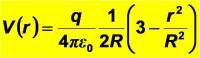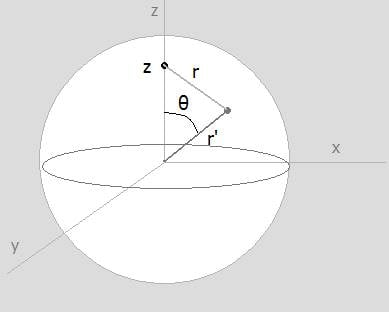# Electric Potential inside insulating solid sphere.

## Homework Statement

Hi everyone,
I'm supposed to find an expression for the electric potential as a function of r, the radial distance inside a solid and non conducting sphere of radius R. A total charge of q is uniformly distributed throughout its volume. The annoying part is that I'm supposed to do that using a relation between the potential and $$\rho$$ , the charge density.

## Homework Equations

The equation the problem wants me to use is:

$$V( \textbf{r} ) = \frac{1}{4 \pi \epsilon_{0}} \int \frac{\rho ( \textbf{r} )}{r} d \tau '$$

$$r$$ being the distance between source & field (observation) point.

I already have obtained a result using a different method (integrating the electric field) and got:This is also what I found on different sources so I'm pretty sure the result is correct.

## The Attempt at a Solution

Yet I can't set up my integration is such a way so as to retrieve this result.
Using the law of cosines on the following figure:I have: $$r^{2} = r'^{2} + z^{2} - 2 r' z cos ( \theta )$$

Using spherical coordinates, I make the integral range from 0 to 2pi and 0 to pi for phi and theta.

For $$r'$$ I am more doubtful. Indeed, when we integrate with respect to the two angles first, we obtain an expression that involves the square root of the following difference: $$(r' - z)^{2}$$.
Thus depending on where the observation point is on the sphere, the positive or negative root is to be taken. So I would guess I could split the integration into two parts, from R (the radius of the sphere) to r, and then from r to 0. Since $$\rho$$ is zero everywhere outside the sphere, there is no need to integrate from infinity, where the potential is assumed to go to zero.

Yet I do not get the expected result, and this using different integration limits. I am assuming I am doing something wrong initially, and if someone has any suggestion I would be very grateful for that.

Last edited:

diazona
Homework Helper
For $$r'$$ I am more doubtful. Indeed, when we integrate with respect to the two angles first, we obtain an expression that involves the square root of the following difference: $$(r' - z)^{2}$$.
In that element $$(r' - z)^{2}$$, $$r'$$ and $$z$$ are simple distances, not vectors, right?
Thus depending on where the observation point is on the sphere, the positive or negative root is to be taken. So I would guess I could split the integration into two parts, from R (the radius of the sphere) to r, and then from r to 0. Since $$\rho$$ is zero everywhere outside the sphere, there is no need to integrate from infinity, where the potential is assumed to go to zero.
Don't you mean from R to z, then z to 0? (or rather, 0 to z and then z to R, I guess)

Subject to the couple of notes above, your setup seems fine. I did the problem setting it up the same way you've described, and I got the expected answer, so perhaps you have a math error somewhere. If you post the details of your work we can help you identify it.

In that element $$(r' - z)^{2}$$, $$r'$$ and $$z$$ are simple distances, not vectors, right?

Don't you mean from R to z, then z to 0? (or rather, 0 to z and then z to R, I guess)

Subject to the couple of notes above, your setup seems fine. I did the problem setting it up the same way you've described, and I got the expected answer, so perhaps you have a math error somewhere. If you post the details of your work we can help you identify it.

Hi diazona,

yes just distances, and yes again for z instead of r, that's what I meant indeed.

I'm going to post here the details of my calculations by Sunday afternoon, thanks a lot for taking a look at my problem.

hi again,

I miraculously realized that I had a math mistake indeed when I was carrying my integration.
I obtained the exact same result, except that I got a minus sign in front of it.
You mentioned that the integration limits should be from 0 to z, then z to R. I did the opposite, which thus gave me the negative of my expected result - yet I'm a bit confused.

Isn't the integration assuming that our reference point is at infinity, thus we're "coming from" infinity, integrating from +∞ to z (or simply R to z since the charge density is zero outside the sphere) and then z to 0. That's what sounds logical to me even though it obviously leads the wrong answer. Do you know why?

diazona
Homework Helper
You're mixing up two completely different equations. The one that requires a reference point at infinity is the definition of V as the path integral of E. When you calculate
$$\int_A^B\vec{E}\cdot\mathrm{d}\vec{s} = V(A) - V(B)$$
all you really get is a difference - that is, you only know that the potential at point A is such-and-such relative to the potential at point B. In order to define "the potential at A" you have to have a standard reference point B.

In this problem, though, the integral defines V as the sum of contributions from each of the individual charge elements that make up the sphere. Conceptually you could think of it as a limit of
$$V = \sum_i V_i = \sum_i \frac{kq_i}{r_i}$$
as the individual charge elements $q_i$ go to zero. There is no path involved here, and what you get isn't a difference between two values, so there's no need to have a standard reference point to compare everything to. The result you get is already absolute.

You're mixing up two completely different equations. The one that requires a reference point at infinity is the definition of V as the path integral of E. When you calculate
$$\int_A^B\vec{E}\cdot\mathrm{d}\vec{s} = V(A) - V(B)$$
all you really get is a difference - that is, you only know that the potential at point A is such-and-such relative to the potential at point B. In order to define "the potential at A" you have to have a standard reference point B.

In this problem, though, the integral defines V as the sum of contributions from each of the individual charge elements that make up the sphere. Conceptually you could think of it as a limit of
$$V = \sum_i V_i = \sum_i \frac{kq_i}{r_i}$$
as the individual charge elements $q_i$ go to zero. There is no path involved here, and what you get isn't a difference between two values, so there's no need to have a standard reference point to compare everything to. The result you get is already absolute.

Oh goodness, I got it. In the second equation we're simply integrating the charge density over the whole sphere while there is no such idea of reference point I was getting myself confused with - we're just adding the "potential" contribution of each individual charge point. Thanks a lot for your help, I really appreciate it.